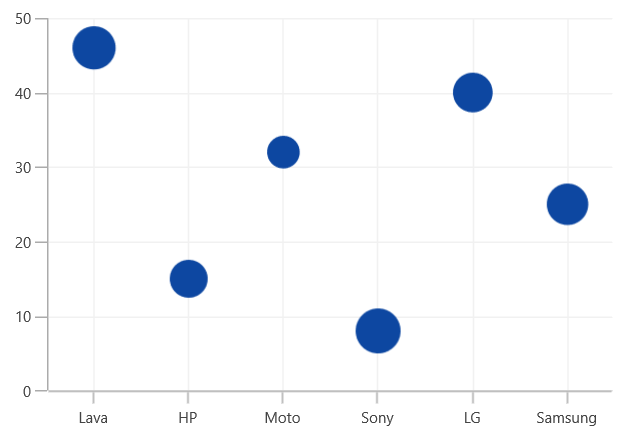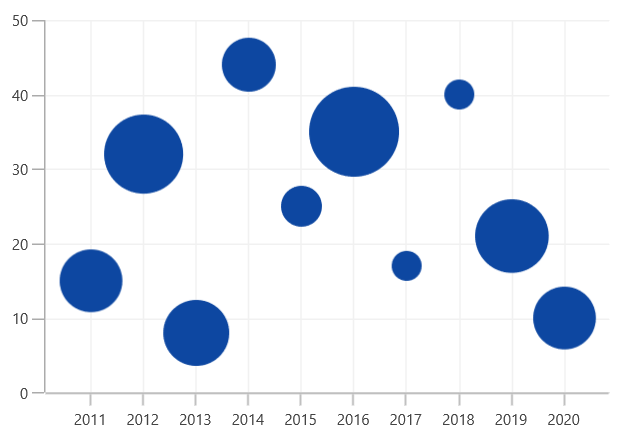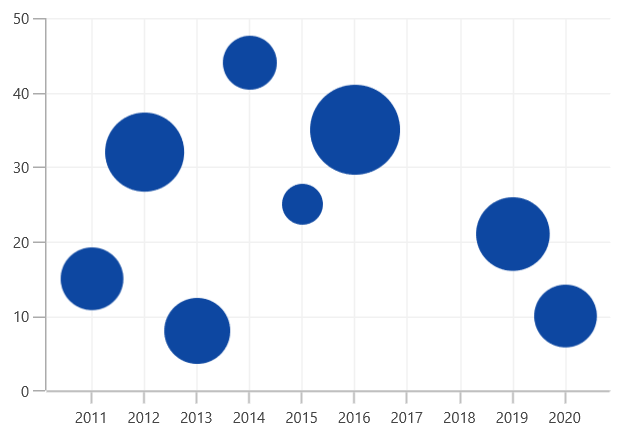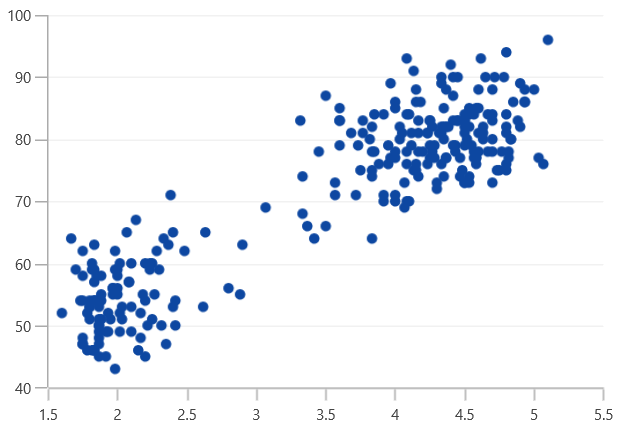# Bubble and Scatter in WinUI Chart

25 May 20222 minutes to read

## Bubble

`BubbleSeries` is represented by closely packed circles, whose areas are proportional to the quantities.

The size of the bubble series is relatively proportional to the value bind with the series using the `Size` property. You can set the constraints on this size using the `MinimumRadius` and `MaximumRadius`.

``<chart:BubbleSeries ItemsSource="{Binding Data}" XBindingPath="XValue" YBindingPath="YValue" Size="Size" MinimumRadius="5" MaximumRadius="10"/>``
``````BubbleSeries series = new BubbleSeries()
{

ItemsSource = new ViewModel().Data,

XBindingPath = "XValue",

YBindingPath = "YValue",

Size = "Size",

};Show Zero Bubbles

The zero size bubble segments can be enabled or disabled by using the `ShowZeroBubbles` property. By default, the property value is true.

The following code illustrates how to set the value to the property.

``````<chart:BubbleSeries ShowZeroBubbles="True">
</chart:BubbleSeries>``````
``````BubbleSeries series = new BubbleSeries();

series.ShowZeroBubbles = true;``````The following code example and screenshots describe when `ShowZeroBubbles` value is false.

``````<chart:BubbleSeries ShowZeroBubbles="False">
</chart:BubbleSeries>``````
``````BubbleSeries series = new BubbleSeries();

series.ShowZeroBubbles = false;``````## Scatter

`ScatterSeries` is similar to bubble series when each point being represented by an ellipse of equal size. This size can be defined by using the `ScatterHeight` and `ScatterWidth` properties.

``<chart:ScatterSeries ScatterHeight="7" ScatterWidth="7" ItemsSource="{Binding Data}" XBindingPath="XValue" YBindingPath="YValue"/>``
``````ScatterSeries series = new ScatterSeries()
{

ItemsSource = new ViewModel().Data,

XBindingPath = "XValue",

YBindingPath = "YValue",

ScatterHeight = 7,

ScatterWidth = 7,

};